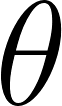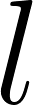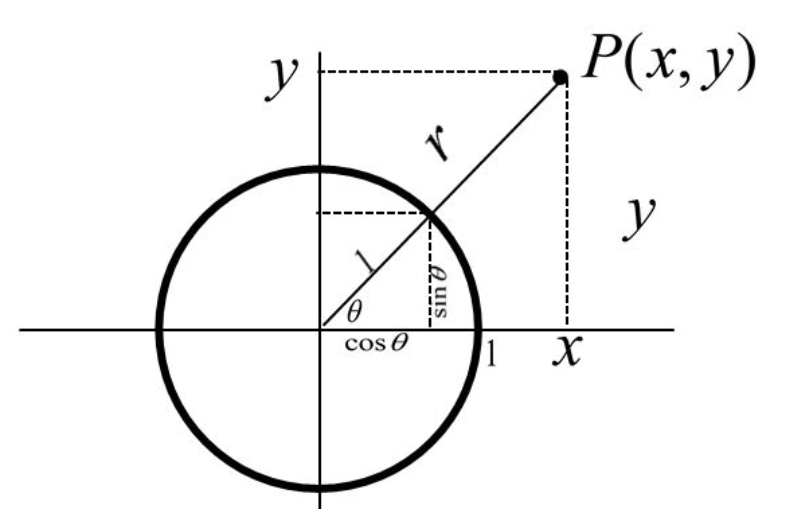×
Get Full Access to Calculus: Early Transcendentals - 1 Edition - Chapter 1.4 - Problem 2e
Get Full Access to Calculus: Early Transcendentals - 1 Edition - Chapter 1.4 - Problem 2e

×

Ch 1.4 - 2EISBN: 9780321570567 2

Solution for problem 2E Chapter 1.4

Calculus: Early Transcendentals | 1st Edition

• Textbook Solutions
• 2901 Step-by-step solutions solved by professors and subject experts
• Get 24/7 help from StudySoup virtual teaching assistantsCalculus: Early Transcendentals | 1st Edition

4 5 1 312 Reviews
22
5
Problem 2E

Explain how a pointon a circle of radiusdetermines an angleand the values of the six trigonometric functions at.

Step-by-Step Solution:

Step 1 of 3

Consider a circle of radiusand a pointat a distancefrom the origin.We need to find the values of the six trigonometric functions at.

Step 2 of 3

Step 3 of 3

ISBN: 9780321570567

Unlock Textbook Solution